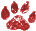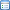# Code Tuning Techniques

 Namespace: VB
How to make FOXPRO applications faster:
There are many ways you can make your Visual FoxPro application faster.

One of the most common structures in a program are the loops. The faster the loops are executed, the faster your program is executed. Switching refers to making a decision inside a loop every time it's executed, which takes processing time. You can unswitch the loop by making the decision once outside the loop rather then every time inside the loop. Here's a Visual foxpro example of a switched loop:
```sumtype=1
netsum=0
grossum=0
cuenta=SECONDS()
FOR i=1 TO 100000
IF sumtype=1
netsum=netsum+i
ELSE
grossum=grossum+i
ENDIF
NEXT
cuentafin=SECONDS()-cuenta
WAIT WINDOW TRANSFORM(cuentafin)
```

Visual foxpro example of an unswitched loop:
```netsum=0
grossum=0
cuenta=SECONDS()
IF sumtype=1
FOR i=1 TO 100000
netsum=netsum+i
NEXT
ELSE
FOR i=1 TO 100000
grossum=grossum+i
NEXT
ENDIF
cuentafin=SECONDS()-cuenta
WAIT WINDOW TRANSFORM(cuentafin)
```

The time savings speak for themselves
 Switched Loop Unswitched Loop Time (example 1) (example 2) Savings 0.272 ms 0.172 ms 36 %I'm not at all sure what this 'proves'.
I guess I could have coded:
```sumtype=1
netsum=0
grossum=0
cuenta=seconds()
if sumtype=1
netsum=netsum + 500005000
else
grossum=grossum + 500005000
endif
cuentafin=seconds()-cuenta
wait window transform(cuentafin)```
and got a result of that isn't even measureable.
While it is axiomatic that one should THINK about what one is coding, I simply do NOT get the point of this exercise. Maybe a real-world example would be more apropos. -- Jim Nelson
Jim -- In the real-world you may be SCANning a table (or DO WHILE !EOF()) and netsum=netsum+i would be netsum=netsum+somefield, and grossum=grossum+i would be grossum=grossum+anotherfield. The example uses a readily available number (the loop variable i) as something it can munch. The point of the exercise is explained in the first paragraph above the example code. HTH, Randy Bosma
I think a similar optimzation that applies to foxpro and loops is to let VFP do the filtering. Perhaps you need to do 'something' to every record in a certain date range. Instead of doing:
```USE MyTable

SCAN
IF BETWEEN(MyTable.TheDate,{01/01/2004},{01/31/2004})
replace MyTable.Posted with .t.
ENDIF
ENDSCAN
```

This should work so much faster:
```USE MyTable

SCAN FOR BETWEEN(MyTable.TheDate,{01/01/2004},{01/31/2004}
replace MyTable.Posted with .t.
ENDSCAN
```

Of course, this is FoxPro... so, this should be the fastest:
```USE MyTable

REPLACE MyTable.Posted WITH .t. FOR BETWEEN(MyTable.TheDate,{01/01/04},{01/31/04})
```

Of course, the second and third will run the fastest if you have an index on "TheDate"

-- Bob Archer

I have to agree, it is a simplistic example. I just neatened it up to be more readable. It does make a fairly useful way to tune an alogrithm or subroutine. Call your code a large, set number of times, tweak it, test again, check for improvements.
```begintime=seconds()
for i=1 to 100000
SubUnderTest()
next
endtime=seconds()-begintime
wait window transform(endtime)
```

Tom Cerul (The VFP newbie)
With this article I only wanted to show a technique (unswitching) you can use in any language
not only in VFP.
Eduardo Díaz GuerreroSee Also: Brute Force Benchmarking, my 1997 DevCon presentation. The Rushmore advice is outdated, but much still applies. -- Ted RocheFor complex performance tuning tasks, a picture can be useful. csnw Perf Logis a free (donations accepted) tool, written in VFP that can capture statistics from VFP code, produce XML reports, and draw SVG images of the processes you are tracking. - lc
Contributors Eduardo Díaz Guerrero, Tom Cerul
Category Code Samples Category VFP Commands Category Performance
( Topic last updated: 2004.04.10 09:30:28 PM )